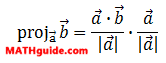Vector Projection
 Home > Lessons > Vector Projection Search | Updated April 9th, 2019
Introduction

This lesson page will explain how to project a vector onto another vector. Here are the sections within this lesson page:

Before continuing with this lesson, it is important that you understand what a vector is and how to calculate magnitude and dot product. Use these lessons before proceeding.esson: Vectorsesson: Vector Magnitudeesson: Dot Product of Vectors

 Projection Formula The formula for calculating the projection of a vector onto another vector is this.The left side of the equation is read, "The projection of vector b onto vector a." The steps for using this formula are demonstrated within the video found in the next section. Instructional Videos This video reveals what the formula is, provides two examples, and explains the graphical representation.ideo: Vector Projection Interactive Quizmasters Try these quizzes to see if you understand the content above.uiz: Vectors: Projection Related Lessons Try these lessons, which contain more information on vectors.esson: Vectorsesson: Engineering Notation (Vectors)esson: Dot Product of Vectorsesson: Unit Vectorsesson: Angle Between Two Vectors